# JP6568979B2 - Design method for number mode optical fiber - Google Patents

## Info

Publication number
JP6568979B2
JP6568979B2 JP2018099856A JP2018099856A JP6568979B2 JP 6568979 B2 JP6568979 B2 JP 6568979B2 JP 2018099856 A JP2018099856 A JP 2018099856A JP 2018099856 A JP2018099856 A JP 2018099856A JP 6568979 B2 JP6568979 B2 JP 6568979B2
Authority
JP
Japan
Prior art keywords
refractive index
core
trench
mode
Prior art date
Legal status (The legal status is an assumption and is not a legal conclusion. Google has not performed a legal analysis and makes no representation as to the accuracy of the status listed.)
Active
Application number
JP2018099856A
Other languages
Japanese (ja)
Other versions
JP2018146973A (en
Inventor

Original Assignee

Priority date (The priority date is an assumption and is not a legal conclusion. Google has not performed a legal analysis and makes no representation as to the accuracy of the date listed.)
Filing date
Publication date
Application filed by 日本電信電話株式会社 filed Critical 日本電信電話株式会社
Priority to JP2018099856A priority Critical patent/JP6568979B2/en
Publication of JP2018146973A publication Critical patent/JP2018146973A/en
Application granted granted Critical
Publication of JP6568979B2 publication Critical patent/JP6568979B2/en
Application status is Active legal-status Critical
Anticipated expiration legal-status Critical

• 239000003365 glass fiber Substances 0 title claims description 105
• 238000005253 cladding Methods 0 claims description 82
• 238000000034 methods Methods 0 claims description 47
• 230000002093 peripheral Effects 0 claims description 45
• 238000005452 bending Methods 0 claims description 31
• 239000000203 mixtures Substances 0 description 7
• 238000004364 calculation methods Methods 0 description 6
• 230000001629 suppression Effects 0 description 3
• 238000005365 production Methods 0 description 2
• 238000004519 manufacturing process Methods 0 description 1

## Images

•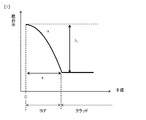•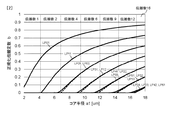•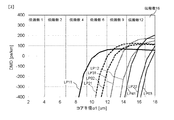•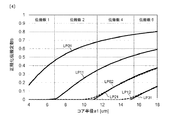•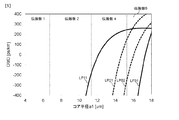•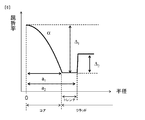•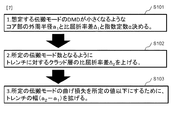•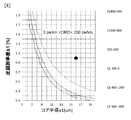•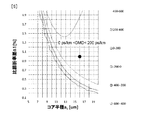•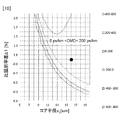•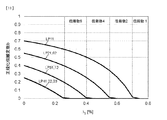•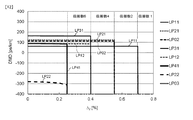•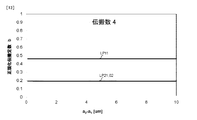•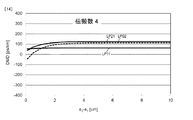•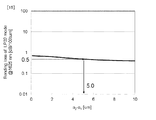•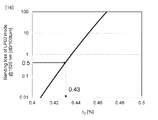••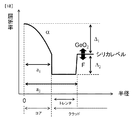•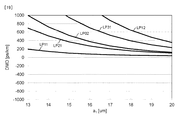•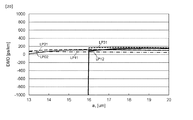••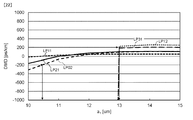•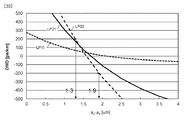•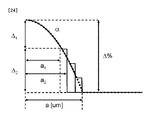•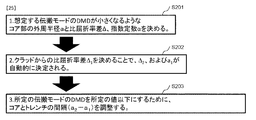•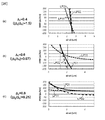•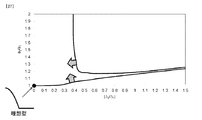•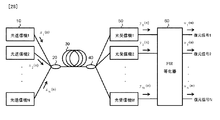## Description

The present invention relates to a number mode optical fiber using a plurality of propagation modes.

A number mode optical fiber using a plurality of propagation modes has been proposed as a technique for expanding the transmission capacity. In particular, mode multiplex transmission using a plurality of propagation modes is attracting attention as a new large-capacity transmission system because the transmission capacity can be improved several times the number of modes.

In transmission using this number mode optical fiber, crosstalk between modes occurs in the transmission path. Therefore, a MIMO (Multiple-Input Multiple-Output) equalizer is used at the receiving end as the compensation means. However, if the group delay difference between the modes (hereinafter referred to as DMD) is large at the receiving end, the load of digital processing (DSP) related to MIMO increases, and in order to realize long-distance transmission, the DSP load is large. Reduction is an issue. Therefore, an optical fiber with a DMD suppressed to about 50 ps / km, an optical fiber having a positive DMD whose fundamental mode is faster than the higher order mode, and an optical fiber having a negative DMD whose fundamental mode is slower than the higher order mode are combined. DMD compensated transmission lines have been proposed (see, for example, Non-Patent Documents 1 and 2).

L. G. -Nielsen, et al. , "Few Mode Transmission Fiber with low DGD, low Mode Coupling and Low Loss", the Proceedings of OFC2012, PDP5A. 1, Mar. 2012. T.A. Sakamoto, T .; Mori, T .; Yamamoto, S .; Tomita, "Differential Mode Delay Managed Transition Line for WDM-MIMO System Using Multi-Step Index Fiber, Journal of LightwaveTyvelwave. 30, no. 17, pp. 2783-2787, September 2012 K. Sato, R.A. Maruyama, N .; Kuwaki, S .; Matsuo, M.M. Ohashi, "Optimized graded index two-mode optical fiber with low DMD, large Aff and low bending loss", Optics Express, vol. 21, no. 14, pp. 16231-16238, Jul. 2013 Ryo Maruyama, Taisaku Shoji, Nobuo Kuwaki, Shoichiro Matsuo, Kiminori Sato, and Masaharu Ohashi, "Design and Fabrication of Long DMD Maximally Flattened Two-Mode Optical Fibres suitable for MIMO Processing", the Proceedings of ECOC2013, Mo. 4). A. 3, Sep. 2013.

However, in Non-Patent Documents 1 and 2, there is a problem that the number of propagation modes is limited to 2, and the number of propagation modes of 3 or more cannot be realized.

Therefore, the present invention has a general graded core configuration (a core whose refractive index decreases exponentially from the central axis toward the outside, and the outside of the core, where the refractive index is smaller than the refractive index of the core, An object of the present invention is to provide a number-mode optical fiber capable of suppressing DMD even if the number of propagation modes is 3 or more by drastically reviewing the configuration of a clad having a uniform refractive index.

In order to achieve the above object, the number mode optical fiber of the present invention has a core structure having a graded refractive index profile and a clad structure in which a trench is disposed in part, and is directed outward from the central axis. The trench (bottom surface) is arranged just outside the core where the refractive index decreases exponentially.

Specifically, the number mode optical fiber according to the present invention is:
A core whose refractive index decreases exponentially from the central axis toward the outside,
A trench disposed outside the core and having a refractive index equal to or lower than the refractive index of the core;
A cladding disposed outside the trench and having a refractive index greater than the refractive index of the trench;
Is provided.

Specifically, the number mode optical fiber according to the present invention is:
A core whose refractive index decreases exponentially from the central axis toward the outside,
A first cladding disposed outside the core and having a refractive index equal to or lower than the refractive index of the core;
A trench disposed outside the first cladding and having a refractive index smaller than that of the first cladding;
A second cladding disposed outside the trench and having a refractive index equal to the first cladding;
With
The refractive index of the first cladding and the second cladding is at a silica level,
The trench includes a dopant that lowers the refractive index below the silica level;
The first cladding simulates the graded shape of the refractive index profile of the core below the silica level up to the trench.

In the number mode optical fiber according to the present invention,
A number mode optical fiber in which at least two number mode optical fibers according to the present invention are cascade-connected,
The two number mode optical fibers propagate four modes, and the absolute values of three DMDs generated between the four mode optical signals are minimized when the index constant of the refractive index of the core is a specific value. ,
One of the two number mode optical fibers has an index constant of the refractive index of the core larger than the specific value,
The other of the two number mode optical fibers may employ a configuration in which the index constant of the refractive index of the core is smaller than the specific value.
Here, the four modes are, for example, LP01, LP21, LP02, and LP11.

Specifically, the optical fiber transmission system according to the present invention is:
A plurality of optical transmitters for converting data into optical signals and transmitting the data;
A multiplexer that multiplexes optical signals from the plurality of optical transmitters so that propagation modes are different, and
A number mode optical fiber according to the present invention for propagating an optical signal from the multiplexer;
A demultiplexer for demultiplexing the optical signal from the several-mode optical fiber at different branching ratios;
A plurality of optical receivers that individually convert each optical signal branched by the duplexer into an electrical signal;
A data restoration unit that compensates for signal degradation that occurs during propagation of the several-mode optical fiber from the electrical signal output by the optical receiver, and restores data transmitted by the plurality of optical transmitters;
Is provided.

Specifically, the design method of the number mode optical fiber according to the present invention is:
A core whose refractive index decreases exponentially from the central axis toward the outside,
A trench disposed outside the core and having a refractive index equal to or lower than the refractive index of the core;
A cladding disposed outside the trench and having a refractive index greater than the refractive index of the trench;
A method for designing a number mode optical fiber comprising:
To calculate DMD (Differential Mode Delay) of each higher-order mode in the used wavelength band when the outer peripheral radius of the core and the relative refractive index difference are changed, so that each higher-order mode DMD satisfies predetermined conditions. A core design procedure for obtaining an exponential constant of the exponential function, an outer peripheral radius of the core and a relative refractive index difference;
Using the outer peripheral radius and relative refractive index difference of the core obtained in the core design procedure, calculate a normalized propagation constant of each higher-order mode in the used wavelength band, and based on the calculated normalized propagation constant, A clad design procedure for determining a range of relative refractive index of the clad with respect to the trench so that each higher-order mode can propagate in
Using the outer peripheral radius and relative refractive index difference of the core determined in the core design procedure and the relative refractive index of the cladding with respect to the trench determined in the cladding design procedure, the bending loss in the used wavelength band was calculated and calculated. A bending loss adjustment procedure for obtaining a width of the trench and a relative refractive index of the clad with respect to the trench so that the bending loss satisfies a predetermined condition;
In order.

Specifically, the design method of the number mode optical fiber according to the present invention is:
A core whose refractive index decreases exponentially from the central axis toward the outside,
A first cladding disposed outside the core and having a refractive index equal to or lower than the refractive index of the core;
A trench disposed outside the first cladding and having a refractive index smaller than that of the first cladding;
A second cladding disposed outside the trench and having a refractive index equal to the first cladding;
With
The refractive index of the first cladding and the second cladding is at a silica level,
The trench includes a dopant that lowers the refractive index below the silica level;
The first cladding is a design method of a number mode optical fiber that simulates the graded shape of the refractive index profile of the core below the silica level up to the trench,
Calculate the DMD of each higher-order mode in the used wavelength band when the outer peripheral radius of the core and the relative refractive index difference are changed, so that each higher-order mode DMD satisfies a predetermined condition. A core ideal value design procedure for obtaining an ideal value of an exponential constant, an outer peripheral radius of the core, and a relative refractive index difference;
The sum of the absolute value of the relative refractive index difference of the first cladding and the second cladding and the absolute value of the relative refractive index difference of the trench is the ideal value of the relative refractive index difference obtained by the core ideal value design procedure. The DMD of each higher order mode obtains a combination of the absolute value of the relative refractive index difference between the first cladding and the second cladding and the absolute value of the relative refractive index difference of the trench satisfying the predetermined condition. Specific refractive index difference design procedure,
For each of the combinations obtained in the relative refractive index difference design procedure, obtain the outer radius of the core using the exponential function at the exponential constant obtained in the core ideal value design procedure, and determine the outer radius of the core. And calculating the DMD of each higher-order mode when the width of the first cladding is changed, and the calculated outer peripheral radius of the core such that the DMD of each higher-order mode satisfies the predetermined condition. A cladding width design procedure for obtaining a range of a ratio of outer radiuses determined by a combination of outer radiuses of the first cladding;
In order.

The above inventions can be combined as much as possible.

According to the present invention, it is possible to provide a number mode optical fiber capable of suppressing DMD even when the number of propagation modes is three or more.

Hereinafter, embodiments of the present invention will be described in detail with reference to the drawings. In addition, this invention is not limited to embodiment shown below. These embodiments are merely examples, and the present invention can be implemented in various modifications and improvements based on the knowledge of those skilled in the art. In the present specification and drawings, the same reference numerals denote the same components.

(Embodiment 1)
The number mode fiber is composed of a core and an outer cladding. The refractive index distribution of the core has an α power refractive index distribution that is expressed by Equation 1 and whose refractive index decreases exponentially from the central axis toward the outside. In Equation 1, n (r) is a refractive index at a position r in the radial direction from the center, n 1 is a refractive index of the core center, Δ 1 is a relative refractive index difference of the core, and α is an exponential constant.

FIG. 1 shows an example of the refractive index distribution of a number mode optical fiber. Refractive index distribution of the number-mode optical fiber, the radius r follows the refractive index profile multiply α is a 1 smaller area. The exponent constant α is a dimensionless parameter indicating a graded profile, and is sometimes called an alpha parameter.

Generally, the outer peripheral radius a 1 of the core part is 25 μm, the relative refractive index difference Δ 1 is 1.0%, α is 2.0, and the number of propagation modes is several tens to hundreds in the communication wavelength band. In order to limit the number of propagation modes, it is necessary to adjust the outer peripheral radius a 1 and the relative refractive index difference Δ 1 of the core. Hereinafter, for the sake of simplicity, the number of propagation modes is converted into an LP mode based on weak waveguide approximation.

Shows the relationship between the outer peripheral radius a 1 and a normalized propagation constant b of the core portion in Fig. In this case, the relative refractive index difference delta 1 is 1.0%, alpha is 2.0, the wavelength is 1550 nm. If you change the outer peripheral radius a 1 of the core portion was calculated normalized propagation constant b based on the finite element method. For the propagation mode, 0 <b <1, and the cutoff condition is expressed as b = 0.

From FIG. 2, for example, when 4.2 [μm] <the outer radius a 1 <6.8 [μm] of the core portion, the number of propagation modes is 2, 6.8 [μm] <the outer radius a 1 <9 of the core portion. An optical fiber with a propagation mode number of 6 can be realized when the propagation mode number is 4, 9.3 [μm] <the outer peripheral radius of the core portion a 1 <11.8 [μm] when .3 [μm].

Next, the case of changing the outer peripheral radius a 1 of the core unit, the high-order mode calculated based on the finite element method (LP11, LP21, LP02, LP31 , LP12, LP41, LP22, LP03 modes) and the fundamental mode (LP01 mode FIG. 3 shows changes in the mode group delay difference (DMD: Differential Mode Delay). DMD is a value obtained by subtracting the group delay of the fundamental mode from the group delay of the higher-order mode, and DMD is calculated at a wavelength of 1550 nm.

FIG. 3 shows that when the LP11 mode is focused, a DMD of 0 [ps / km] can be realized when the core radius a1 is 10.2 [μm]. However, the two-mode operation cannot be realized. Similarly, even when attention is paid to higher-order modes than the LP11 mode, it is difficult to realize a low DMD while limiting the number of propagation modes.

On the other hand, there is a report that realizes a low DMD with two propagation modes by optimizing the relative refractive index differences Δ 1 and α of the structural parameters (for example, Non-Patent Document 3). 4 to the relative refractive index difference delta 1 is 0.4%, alpha is 2.3, the wavelength showing the relationship between the core radius a1 and the normalized propagation constant b for the time of 1550 nm. From FIG. 4, it is possible to realize an optical fiber having a propagation mode number of 2 when 6.5 [μm] <the outer peripheral radius of the core portion a 1 <11.2 [μm].

Next, the case of changing the outer peripheral radius a 1 of the core unit, the high-order mode calculated based on the finite element method (LP11, LP21, LP02, LP31 mode) and the mode group delay difference of the fundamental mode (LP01 mode) (DMD : Differential Mode Delay) is shown in FIG. FIG. 5 shows that when the LP11 mode is focused, a DMD of −200 [ps / km] can be realized when the outer peripheral radius a 1 of the core portion is 11.2 [μm]. Two-mode operation can also be realized. However, even when attention is paid to higher-order modes than the LP11 mode, it can be seen that it is difficult to realize a low DMD while limiting the number of propagation modes.

That is, when the number of propagation modes having a mode number greater than 2 is taken into consideration, the graded core alone has a low DMD (−200 [ps / km] <DMD <200 [ps / km] even if the structural parameters are adjusted. ) And N-mode operation (N> 2) is difficult to realize.

The manufacturing error of a typical core radius is ± 0.25 μm, and the absolute value of DMD is set to 200 ps / km or less as a target value in the 2LP mode. Even if the design value of the DMD is 0 ps / km, the DMD cannot be strictly set to 0 for fiber fabrication, and the DMD changes within a range of at least ± 200 ps / km even if severe manufacturing conditions are assumed. . Therefore, also in the optical fiber of this embodiment, the target value is ± 200 ps / km or less.

FIG. 6 is a diagram showing a refractive index distribution in the radial direction in the number mode optical fiber of the present embodiment. The number mode optical fiber has a core from the center to the radius a 1 , a cladding outside the core, and a trench formed in the cladding. Here, the trench is a relatively low refractive index region of the clad, and is formed in a region from a 1 to a 2 (outside of the core having α power refractive index distribution) in FIG.

Refractive index distribution of the number-mode optical fiber of this embodiment, the radius r is a 1 smaller area, according to the α-th power refractive index distribution represented by the number 1. The core is a graded type whose refractive index profile in the radial direction from the center has an exponential constant α. The relative refractive index of the center of the core relative to the trench is delta 1. The relative refractive index Δ 2 of the cladding layer with respect to the trench. The refractive index profile of the trench is preferably lower and uniform than the cladding.

Hereinafter, a design method of a low DMD number mode optical fiber having a propagation mode number larger than 2 will be described in detail with reference to FIG. The design method of the number mode optical fiber according to the present embodiment includes a core design procedure (S101), a cladding design procedure (S102), and a trench design procedure (S103) in this order. As an example, an optical fiber having a propagation mode of 4 (LP01, LP11, LP21, LP02 mode) and a DMD of 200 ps / km or less is a design target. The wavelength is considered at 1550 nm.

First, perform the core design procedure (S101), determining the outer circumference radius a 1 and a relative refractive index difference delta 1 and exponent constant α of the core portion. For example, the DMD of each higher-order mode in the used wavelength band when the outer peripheral radius a 1 and the relative refractive index difference Δ 1 are changed is calculated so that each higher-order mode DMD satisfies a predetermined condition. Then, the outer peripheral radius a 1 and the relative refractive index difference Δ 1 of the core are obtained.

In the present embodiment, the used wavelength band is 1550 nm, and the predetermined condition is 200 ps / km or less. 8 to 10 show examples of DMDs of higher order modes when the outer peripheral radius a 1 and the relative refractive index difference Δ 1 of the core part are changed. 8 shows the LP11 mode, FIG. 9 shows the LP21 mode, and FIG. 10 shows the LP02 mode. In the present embodiment, α is set to 2.0 as an example in the calculation of DMD. 8 to 10, when the outer peripheral radius a 1 of the core portion is 16 [μm] and the relative refractive index difference Δ 1 is 1.0 [%], in all modes of LP11, LP21, and LP02. DMD is 0 to 200 ps / km. Therefore, as the structural parameters, the outer peripheral radius a 1 of the core portion is set to 16 [μm], and the relative refractive index difference Δ 1 is set to 1.0 [%].

Then run clad design procedure (S102), raising the relative refractive index delta 2 of the clad layer with respect to the trench. For example, the normalized propagation constant b of each higher-order mode in the used wavelength band is calculated using the core outer radius a 1 and the relative refractive index difference Δ 1 obtained in the core design procedure. Based on the calculation result of the normalized propagation constant b, the range of the relative refractive index Δ2 of the cladding with respect to the trench is obtained so that each higher-order mode can propagate in the used wavelength band.

In the present embodiment, the outer peripheral radius a 1 of the core portion is 16 [μm], the relative refractive index difference Δ 1 is 1.0 [%], and the used wavelength band is 1550 nm. The normalized propagation constant b in the case of changing the relative refractive index delta 2 of the clad layer for the trench shown in FIG. 11. The width (a2-a1) of the trench was 7 [μm], and the wavelength was 1550 [nm]. FIG. 11 shows that the number of propagation modes is 4 when 0.40 <Δ 2 <0.55 [%].

Here, FIG. 12 shows a DMD when the relative refractive index Δ 2 of the cladding layer with respect to the trench is changed. FIG. 12 shows that DMD is not affected by the relative refractive index Δ 2 of the cladding layer with respect to the trench.

FIG. 13 shows the normalized propagation constant b and FIG. 14 shows the DMD for the trench width (a2-a1). At this time, delta 2 was 0.45%. 13 and 14, it can be seen that the trench width (a2-a1) has little influence on the number of propagation modes and DMD.

Next, in order to adjust the bending loss of the propagating mode, perform the trench design procedure (S103), fine adjustment of the relative refractive index delta 2 of the clad layer to the width (a2-a1) and the trench of the trench. For example, using the range of the outer peripheral radius a 1 and the relative refractive index difference Δ 1 obtained in the core design procedure and the relative refractive index Δ 2 of the clad layer with respect to the trench obtained in the clad design procedure, the bending loss in the used wavelength band Is calculated. Then, a value is selected from the range of the relative refractive index Δ2 of the cladding layer with respect to the trench so that the calculated bending loss satisfies a predetermined condition, and the width (a2-a1) of the trench is obtained.

In the present embodiment, the outer peripheral radius a 1 of the core portion is 16 [μm], the relative refractive index difference Δ 1 is 1.0 [%], and the range of the relative refractive index Δ 2 is 0.40 <Δ 2 <0.55. % And the wavelength band used is 1550 nm. For example, in the case of a single-mode cutoff shift fiber (ITU-T G.654), the bending loss at a bending radius of 30 mm is 0.5 dB / 100 turn or less in the wavelength band used.

Here, the calculation of the bending loss of the propagation mode may be made by considering the LP02 mode in which the bending loss is the largest among the propagation modes. Further, assuming the use in the C + L band (1530 to 1625 nm), the calculation of the bending loss in the propagation mode needs to consider 1625 nm at which the bending loss becomes the largest.

FIG. 15 shows the LP02 mode bending loss when the trench width (a2-a1) is changed. At this time, the relative refractive index Δ 2 of the cladding layer with respect to the trench was set to 0.43 [%]. Figure 16 shows the LP02 mode bending loss when changing the relative refractive index delta 2 of the clad layer with respect to the trench. At this time, the width (a2-a1) of the trench was set to 10 [μm]. From FIG. 15, it can be seen that the bending loss can be finely adjusted by the width (a2-a1) of the trench. FIG. 16 shows that the bending loss can be largely adjusted by the relative refractive index Δ 2 of the cladding layer with respect to the trench.

In all propagation modes, in order to obtain a bending loss of 0.5 dB / 100 turn or less at a bending radius of 30 mm, the trench width (a2-a1) is 5.0 [μm] or more, and the relative refractive index of the cladding layer with respect to the trench delta 2 height 0.43 [%] may be any less. Furthermore, when cut-off is considered, 0.4 [%] or more is desirable.

FIG. 17 shows changes in DMD between the higher-order modes (LP11, LP21, LP02) and the basic mode (LP01 mode) calculated based on the finite element method when the exponent constant α of the core portion is changed. As shown in FIG. 17, when the exponent constant α of the core is 1.92 to 2.02, the absolute value of DMD is 200 ps / km or less in all higher-order modes. When α is 1.97, DMD is the smallest.

By setting each structural parameter of the refractive index distribution by the above procedure, a number mode optical fiber having a propagation mode number of 4 and an DMD absolute value of 200 ps / km or less can be manufactured. This design method is effective even when the number of propagation modes is larger than four.

As described above, the number mode optical fiber according to the present embodiment includes the core whose refractive index decreases exponentially from the central axis toward the outside, the outside of the core, and the refractive index is the refractive index of the core. And a trench that is between the core and the cladding and has a refractive index that is less than or equal to the refractive index of the cladding. The core decreases exponentially to the trench. And by making these parameters of the refractive index distribution appropriate, the number of propagation modes can be made larger than 2, and the group delay difference between the propagation modes can be reduced. Therefore, the invention according to the present embodiment can provide a number mode optical fiber and an optical fiber transmission system that can reduce the group delay difference between the propagation modes and can increase the number of propagation modes to more than two.

(Embodiment 2)
For example, in the number mode optical fiber of this embodiment, at least two of the number mode optical fibers described in the first embodiment are connected in cascade.

In the two number mode optical fibers, the higher order mode DMD is minimized when the index constant of the refractive index of the core is a specific value. For example, in the case of the number mode optical fiber according to Embodiment 1 shown in FIG. 17, the specific value is α = 1.97.

One of the two number mode optical fibers has an index constant α of the refractive index of the core larger than a specific value of 1.97. In the other of the two number mode optical fibers, the index constant α of the refractive index of the core is smaller than the specific value 1.97.

As described above, the number mode optical fiber of the present embodiment is characterized in that the core exponent constant α is different in the section in the light propagation direction. Furthermore, when there are two or more sections, the exponent constant α of at least one section is 1.97 or more, and the exponent constant α of at least one section is less than 1.97.

From the graph of FIG. 17, the DMDs of the second higher order mode and the third higher order mode are almost equal and grouped, and the DMD increases from negative to positive at the core constant α = 1.97. You can see how it is. Furthermore, the DMD of each higher order mode is proportional to the exponent constant α of the core. As a result, even when it is difficult to reduce the DMD using a single optical fiber due to manufacturing errors, it is possible to further reduce the DMD by connecting the positive and negative optical fibers and adjusting the fiber length. .

For example, mode delay compensated transmission with a propagation mode number of 4 by connecting a 4-mode fiber having a negative DMD of α = 1.85 and a 4-mode fiber having a positive DMD of α = 2.10 by the same fiber length. A road can be realized. At this time, DMD can be 100 ps / km or less.

As described above, the mode group delay difference of the number mode optical fiber can be adjusted to be positive or negative by adjusting each parameter of the refractive index distribution, and the positive mode group delay difference of the number mode optical fiber and the negative mode group By connecting to a delay mode number mode optical fiber, it is possible to manufacture a number mode optical fiber (mode delay compensation transmission line) having a propagation mode number of 4 and a mode group delay difference of 100 ps / km or less.

By adopting the design method of the first embodiment, it is possible to realize a mode delay compensation transmission line when the number of propagation modes is larger than four.

(Embodiment 3)
In the case of manufacturing the optical fiber having the refractive index profile of FIG. 6 shown in the first embodiment, there is a technique in which the trench is silica, and the core portion and the cladding portion are doped with germanium oxide (GeO 2) to increase the refractive index. I think that it is general (for example, refer nonpatent literature 4). However, since the amount of doping into the core and cladding is increased, it is considered that the difficulty in manufacturing and the increase in the manufacturing cost become problems. Therefore, a means is conceivable in which the cladding layer is made to be a silica level and the trench is doped with fluorine (F) to be lower than the silica level. FIG. 18 shows this refractive index distribution.

In the refractive index distribution shown in FIG. 18, a core having a graded profile, a cladding outside the core and having a refractive index smaller than the refractive index of the core, and between the core and the cladding, the refractive index is clad. And a trench having a refractive index equal to or lower than the above. The core has an outer radius of a 1 , a relative refractive index relative to the cladding layer of Δ 1 , and an exponential constant of α. It is assumed that the trench has a radius to the outer boundary of the trench of a 2 and a relative refractive index of Δ 2 with respect to the cladding layer.

When each parameter of the refractive index distribution is a 2 −a 1 = 11 μm, Δ 1 = 0.6%, Δ 2 = −0.4%, α = 2.0, and the core part outer radius a 1 is changed The DMD is shown in FIG. From FIG. 19, it can be seen that the refractive index profile in FIG. 18 cannot limit the number of propagation modes and reduce DMD.

For reference, each parameter of the refractive index distribution in the refractive index distribution of FIG. 6 shown in Embodiment 1 is a 2 −a 1 = 7 μm, Δ 1 = 1.0%, Δ 2 = 0.45%, α = and 2.0, showing the DMD when changing the core outer circumference radius a 1 in FIG. 20. In the refractive index profile of FIG. 6 shown in Embodiment 1, it can be seen that the number of propagation modes can be limited and DMD can be reduced.

Compared with the refractive index distribution of FIG. 6, the refractive index distribution of FIG. 18 does not maintain a graded shape up to the trench, and therefore it is considered that DMD has a large value.
Therefore, the above problem is solved by the refractive index distribution of FIG.

For example, each parameter of the refractive index distribution includes a core-trench distance a 2 −a 1 = 1.5 μm, a trench width a 3 −a 2 = 11 μm, and Δ 1 = 0. FIG. 22 shows a DMD when 6%, Δ 2 = −0.4%, α = 2.0, and the outer peripheral radius a 1 of the core is changed. FIG. 22 shows that a DMD equivalent to that in FIG. 20 is obtained. If the outer peripheral radius a 1 of the core portion of 10.4～13.0Myuemu, it can be seen the absolute value of the number of propagation modes 4 and the DMD is 200 ps / miles or less of the optical fiber can be realized. As described above, by providing the interval (a 2 −a 1 ) between the core and the trench, it is possible to simulate the graded shape as if the trench.

First, the core portion outer radius a 1 = 12.0 μm, Δ 1 = 0.6%, Δ 2 = −0.4%, α = 2.0 are fixed, and the interval between the core and the trench a 2 −a 1 Consider the case of changing. FIG. 23 shows a DMD when the interval a 2 -a 1 between the core and the trench is changed. From FIG. 22, when the distance a 2 -a 1 between the core and the trench is 1.3 to 1.9 μm, the absolute value of DMD becomes 200 ps / km or less in all higher-order modes (LP11, LP21, LP02 modes). If the distance between the core and the trench becomes too large, it is considered that the graded shape cannot be simulated. As described above, by adjusting the interval a 2 -a 1 between the core and the trench, the DMD can be reduced by the four-mode operation.

Next, consider a case where the silica level is changed.
Core radius a, the relative refractive index difference to be ideal in Figure 24 delta, graded profile and exponential constants alpha, showing the profile of the optical fiber proposed in the case of changing the ratio of delta 1 and delta 2. At this time, | Δ 1 | + | Δ 2 | = Δ.

Hereinafter, a design method of a low DMD number mode optical fiber having a propagation mode number larger than 2 will be described in detail with reference to FIG. The design method of the number mode optical fiber according to the present embodiment includes a core ideal value design procedure (S201), a relative refractive index difference design procedure (S202), and a cladding width design procedure (S203) in this order. As an example, an optical fiber having a propagation mode of 4 (LP01, LP11, LP21, LP02 mode) and a DMD of 200 ps / km or less is a design target. The wavelength is considered at 1550 nm.

First, the core ideal value design procedure (S201) is executed, and the outer peripheral radius a, the relative refractive index difference Δ, and the exponent constant α are determined so that the DMD of the assumed propagation mode becomes small. For example, the DMD of each higher-order mode in the wavelength band to be used when the outer peripheral radius “a” and the relative refractive index difference Δ are changed is calculated so that each higher-order mode DMD satisfies a predetermined condition. The ideal value a of the outer peripheral radius and the ideal value Δ of the relative refractive index difference are obtained.

In the present embodiment, the used wavelength band is 1550 nm, and the predetermined condition is 200 ps / km or less. As shown in the first embodiment, the ideal value a of the outer peripheral radius of the core portion is set to 16 [ μm], the ideal value Δ of the relative refractive index difference is 1.0 [%], and the exponential constant α is 2.0.

Then, run the relative refractive index difference design procedure (S202), by determining the relative refractive index difference delta 1 from clad, Delta] 2, and a 1 are determined automatically. From Equation 1, if the values of Δ / a a are equal, the shape is the same from the center to a 1 . For example, the relative refractive index difference of the cladding | delta 1 | and the relative refractive index difference of the trench | so that the sum of the ideal value delta relative refractive index difference obtained in the core ideal value design procedure, | | delta 2 delta 1 | And | Δ 2 | are obtained. As a result, the outer peripheral radius a1 of the core can be calculated using the α power refractive index distribution function of Equation 1.

In the present embodiment, the ideal value a of the outer peripheral radius of the core portion is 16 [μm], and the ideal value Δ of the relative refractive index difference is 1.0 [%]. In the case of Δ 1 = 0.4 [%], Δ 2 = −0.6 [%], and | Δ 2 / Δ 1 | = 1.5. a 1 is determined to be 10.1 [μm] from Equation 1. When Δ 1 = 0.6 [%], Δ 2 = −0.4 [%], and Δ 2 / Δ 1 = 0.67. a 1 is determined to be 12.4 [μm] from Equation 1. When Δ 1 = 0.8 [%], Δ 2 = −0.2 [%], and Δ 2 / Δ 1 = 0.25. a 1 is determined to be 14.3 [μm] from Equation 1.

26A, Δ 1 is 0.4 [%], Δ 2 is −0.6 [%], | Δ 2 / Δ 1 | is 1.5, and the outer peripheral radius a 1 of the core portion is 10. The DMD is shown when the distance a 2 -a 1 between the core and the trench is changed while being fixed at 1 [μm]. From FIG. 26A, the absolute value of the DMD is obtained in all higher-order modes (LP11, LP21, LP02 modes) when the interval a 2 -a 1 between the core and the trench is 2.4 to 2.6 [μm]. 200 ps / km or less. In this case, the range of a 2 / a 1 is 1.24 to 1.26.

In FIG. 26B, Δ 1 is 0.6 [%], Δ 2 is −0.4 [%], | Δ 2 / Δ 1 | is 0.67, and the outer peripheral radius a 1 of the core is 12. The DMD is shown when the distance a 2 -a 1 between the core and the trench is changed while being fixed at 4 [μm]. From FIG. 26B, the absolute value of DMD is 200 ps / km in all higher-order modes (LP11, LP21, LP02 modes) when the distance a 2 -a 1 between the core and the trench is 1.3 to 2.1 μm. It becomes the following. At this time, the range of a 2 / a 1 is 1.10 to 1.17.

Figure 26 (c), Δ 1 to 0.8%, delta 2 to -0.2%, | Δ 2 / Δ 1 | 0.25, secure the outer peripheral radius a 1 of the core portion to 14.3μm The DMD when the distance a 2 -a 1 between the core and the trench is changed is shown. From FIG. 26 (c), the absolute value of DMD becomes 200 ps / km or less in all higher-order modes (LP11, LP21, LP02 modes) when the distance a 2 -a 1 between the core and the trench is 0.1 or more. . At this time, the range of a 2 / a 1 is 1.01 or more.

Thus, | Δ2 / Δ1 | is changed (Δ 1 : variable, Δ 2 : fixed), and the absolute value of DMD becomes 200 ps / km or less, a 2 / a 1 (a 1 : fixed, a 2 : variable) 27), the design range shown in FIG. 27 is obtained. A region surrounded by two curves is a region where the absolute value of DMD is 200 ps / km or less when the number of propagation modes is four.

From Embodiment 1, the DMD is not affected by the relative refractive index of the cladding layer with respect to the trench. The trench width has almost no influence on the number of propagation modes and DMD. Therefore, also in this embodiment, the DMD can be controlled by designing only the core portion | Δ2 / Δ1 | and a 2 / a 1 .

By setting each structural parameter of the refractive index distribution by the above procedure, a number mode optical fiber having a propagation mode number of 4 and an absolute value of DMD of 200 ps / km or less can be manufactured. Although there are six structural parameters, by adjusting only Δ1 and a 2 by the above procedure, only a predetermined number of modes can be easily propagated, and a low-DMD number-mode optical fiber can be realized. This design method is effective even when the number of propagation modes is larger than four.

(Embodiment 4)
FIG. 28 is a schematic diagram of the optical fiber transmission system of the present embodiment. Optical fiber transmission system
N (N is an integer of 2 or more) optical transmitters 10 each transmitting N data as optical signals,
A multiplexer 20 that multiplexes N optical signals from the optical transmitter 10 in different propagation modes;
A number mode optical transmission line 30 for propagating an optical signal from the multiplexer 20;
A demultiplexer 40 for demultiplexing the optical signal from the number mode optical transmission line 30 at different branching ratios;
M optical receivers 50 (M is an integer equal to or greater than N) for receiving optical signals from the duplexer 40;
As a data restoration unit that compensates for signal degradation caused during propagation in the several-mode optical transmission line 30 from M electrical signals output from the optical receiver 50 and restores N data transmitted by the optical transmitter 10 A functioning FIR equalizer 60;
Is provided.

N types of signals (x (n)) emitted from N (N is an integer of 2 or more) transmitters 10 are combined such that the propagation mode ratios to be combined in the multiplexer 20 are different. The propagation mode ratio is the ratio of the intensity of each propagation mode that propagates through the number mode optical transmission line 30. The number mode optical transmission line 30 is an optical transmission line using the number mode optical fiber described in the first or third embodiment. As described in the second embodiment, the number mode optical transmission line 30 may use a number mode optical fiber having a DMD compensation function by cascading a plurality of number mode optical fibers having different α. In this case, the number mode optical fiber connected in cascade may be the number mode optical fiber of the first embodiment or the number mode optical fiber of the third embodiment. In consideration of the degree of restoration of the transmitted signal, it is desirable that the propagation mode ratio is greatly different for each optical signal. For example, it is desirable that the multiplexer 20 has a three-dimensional waveguide structure that can excite any mode by changing the location where light is applied to the fiber core.

The multiplexed optical signal enters the several-mode optical transmission line 30 and is demultiplexed into M ports (M is an integer equal to or greater than N) with a different branching ratio in a duplexer 40 installed on the output side. Regarding the branching ratio, similarly to the multiplexer 20, the optical signal is branched so that all the propagation modes do not have the same ratio. When considering the degree of signal restoration, it is desirable that the branching ratio differs greatly from port to port.

The demultiplexed M kinds of signals (y (n)) are received by the M receivers 50, and the FIR equalizer 60 installed in the subsequent stage compensates for the signal deterioration received by the several mode optical fiber and restores it. A signal (u (n)) is obtained. The optical fiber transmission system is N-input M-output MIMO transmission, and N types of signals can be transmitted in parallel.
The FIR equalizer 60 can also compensate for mode dispersion, chromatic dispersion, and polarization dispersion. Further, in order to acquire the electric field amplitude and phase information of the received signal, the receiver 50 is configured by a local light source, a 90 ° optical hybrid, a balance receiver, an analog-digital converter, and a calculator.

The FIR equalizer 60 can compensate for the linear distortion generated in the number mode optical transmission line 30, and is generated in the number mode optical transmission line 30 by appropriately setting the delay amount and coefficient of the tap. It is possible to compensate for signal degradation caused by interference from other transmitters, mode dispersion, chromatic dispersion, and polarization dispersion. However, when compensating for signal degradation due to mode dispersion, if the difference in mode group delay between the basic mode and the higher-order mode becomes large, the amount of calculation required for signal degradation compensation becomes enormous. However, the optical fiber transmission system uses the number mode optical transmission line 30 having a small mode group delay difference, and the amount of calculation required for compensation can be reduced. Furthermore, since the number of propagation modes is larger than 2, the capacity can be increased. In other words, by providing the several-mode optical transmission line 30, it is possible to increase the capacity and distance of the transmission system with the conventional FIR equalizer.

As described above, the present optical fiber transmission system includes the number mode optical fiber that can reduce the group delay difference in the light propagation mode, and can reduce the load of digital signal processing during reception. Therefore, the invention according to this embodiment can provide an optical fiber transmission system that can reduce the load of digital processing necessary for signal restoration.

The present invention can realize a large capacity and a long distance of optical fiber transmission by using a higher-order mode in the fiber.

10: optical transmitter 20: multiplexer 30: number mode optical transmission line 40: demultiplexer 50: optical receiver 60: FIR equalizer

## Claims (1)

1. A core whose refractive index decreases exponentially with an exponential constant α from the central axis toward the radius a1 toward the outside;
A first cladding disposed outside the core, having a uniform refractive index at radii a1 to a2 and a refractive index equal to the refractive index of the core at a radius a1 ;
A trench disposed outside the first cladding and having a uniform refractive index at radii a2 to a3 and smaller than the refractive index of the first cladding;
A second cladding disposed outside the trench and having a refractive index equal to the first cladding;
With
The relative refractive index difference between the center of the core and the first cladding is Δ1,
The relative refractive index difference between the first cladding and the trench is Δ2,
The refractive index of the first cladding and the second cladding is at a silica level,
The trench includes a dopant that lowers the refractive index below the silica level;
The sum of absolute values of Δ1 and Δ2 is a relative refractive index difference Δ,
Δ1, Δ2, a1, a2 so that the core decreases exponentially from the central axis and the refractive index distribution shape in which the relative refractive index difference becomes Δ at the outer peripheral radius a is simulated by the first cladding and the trench. And a3 , a number mode optical fiber design method comprising:
An exponent of the exponential function is calculated so as to calculate a DMD of an assumed propagation mode in a used wavelength band when the outer peripheral radius a of the core and the relative refractive index difference Δ are changed, and each higher-order mode DMD satisfies a predetermined value. A core ideal value design procedure for obtaining an ideal value of a constant α, an outer peripheral radius a of the core, and a relative refractive index difference Δ;
A relative refractive index difference design procedure in which the sum of the absolute value of Δ1 and the absolute value of Δ2 is an ideal value of the relative refractive index difference Δ obtained by the core ideal value design procedure;
In the refractive index distribution when Δ1 obtained in the relative refractive index difference design procedure and the exponential constant α obtained in the core ideal value design procedure, the radius a1 of the refractive index that becomes Δ−Δ1 is expressed by The DMD of each higher-order mode when the width of the first cladding is changed using the radius a1 is calculated, and the calculated DMD of each higher-order mode satisfies the predetermined condition. A cladding width design procedure for determining the radius a1 and the radius a2;
Using Δ1, Δ2, a1, a2 obtained in the relative refractive index difference design procedure and the cladding width design procedure, the bending loss is adjusted by the trench width a3 so that only a predetermined number of modes propagate in the used wavelength band. , Trench width design procedure ,
A method of designing a number mode optical fiber having
(Equation 1)

Where r is the radial distance from the center,
n (r) is the refractive index at a distance r in the radial direction from the center,
n1 is the refractive index of the core center,
And
JP2018099856A 2018-05-24 2018-05-24 Design method for number mode optical fiber Active JP6568979B2 (en)

## Priority Applications (1)

Application Number Priority Date Filing Date Title
JP2018099856A JP6568979B2 (en) 2018-05-24 2018-05-24 Design method for number mode optical fiber

## Applications Claiming Priority (1)

Application Number Priority Date Filing Date Title
JP2018099856A JP6568979B2 (en) 2018-05-24 2018-05-24 Design method for number mode optical fiber

## Related Parent Applications (1)

Application Number Title Priority Date Filing Date
JP2014095098 Division 2014-05-02

## Publications (2)

Publication Number Publication Date
JP2018146973A JP2018146973A (en) 2018-09-20
JP6568979B2 true JP6568979B2 (en) 2019-08-28

# Family

## Family Applications (1)

Application Number Title Priority Date Filing Date
JP2018099856A Active JP6568979B2 (en) 2018-05-24 2018-05-24 Design method for number mode optical fiber

## Country Status (1)

JP (1) JP6568979B2 (en)

## Family Cites Families (5)

* Cited by examiner, † Cited by third party
Publication number Priority date Publication date Assignee Title
JPH1164665A (en) * 1997-06-13 1999-03-05 Sumitomo Electric Ind Ltd Optical fiber
JP5330729B2 (en) * 2008-04-16 2013-10-30 三菱電線工業株式会社 Graded index multimode optical fiber
US8670643B2 (en) * 2011-05-18 2014-03-11 Corning Incorporated Large effective area optical fibers
EP2817665B1 (en) * 2012-02-20 2018-12-12 Corning Incorporated Mode delay managed few moded optical fiber link
US8948559B2 (en) * 2012-09-05 2015-02-03 Ofs Fitel, Llc Multiple LP mode fiber designs for mode division multiplexing

## Also Published As

Publication number Publication date
JP2018146973A (en) 2018-09-20

## Similar Documents

Publication Publication Date Title
US9088364B1 (en) Optical transmission system and multi-core optical fiber
JP2016103031A (en) LARGE EFFECTIVE AREA FIBER WITH Ge-FREE CORE
US8285094B2 (en) Multicore fiber
JP2016122209A (en) Multicore fiber design for spatial multiplexing
JP5732234B2 (en) High-band multimode optical fiber
CN101738688B (en) Multimode optical system
US8971682B2 (en) Few mode optical fibers
US6470126B1 (en) Dispersion compensating optical fiber, and wavelength division multiplexing transmission line using a dispersion compensating optical fiber
KR100728452B1 (en) Dispersion Shifted Fiber
Hayashi et al. Design and fabrication of ultra-low crosstalk and low-loss multi-core fiber
EP1255138B1 (en) Positive dispersion optical fiber having large effective area
US8041172B2 (en) Transmission optical fiber having large effective area
US8948559B2 (en) Multiple LP mode fiber designs for mode division multiplexing
US7406237B2 (en) Multiband optical fiber
US5838867A (en) Dispersion compensating fiber and optical transmission system including the same
US6169837B1 (en) Dispersion-flattened optical fiber
EP1067412B1 (en) Dispersion compensating optical fiber
JP5606742B2 (en) Single mode optical fiber
DE60024158T2 (en) Optical fiber for wavelength division multiplex transmissions
US20110222828A1 (en) Multi-core optical fiber
JP5324012B2 (en) Multi-core optical fiber and optical transmission system
US6879764B2 (en) Dispersion shifted fiber having low dispersion slope
JP5426411B2 (en) Single mode optical fiber with increased effective area
JP2012203416A (en) Multimode optical fiber with improved bend resistance
JP2017526960A (en) Low loss fumode fiber (FMF)

## Legal Events

Date Code Title Description
A621 Written request for application examination

Free format text: JAPANESE INTERMEDIATE CODE: A621

Effective date: 20180524

A131 Notification of reasons for refusal

Free format text: JAPANESE INTERMEDIATE CODE: A131

Effective date: 20190228

A977 Report on retrieval

Free format text: JAPANESE INTERMEDIATE CODE: A971007

Effective date: 20190227

A521 Written amendment

Free format text: JAPANESE INTERMEDIATE CODE: A523

Effective date: 20190418

TRDD Decision of grant or rejection written
A01 Written decision to grant a patent or to grant a registration (utility model)

Free format text: JAPANESE INTERMEDIATE CODE: A01

Effective date: 20190730

A61 First payment of annual fees (during grant procedure)

Free format text: JAPANESE INTERMEDIATE CODE: A61

Effective date: 20190805

R150 Certificate of patent or registration of utility model

Ref document number: 6568979

Country of ref document: JP

Free format text: JAPANESE INTERMEDIATE CODE: R150